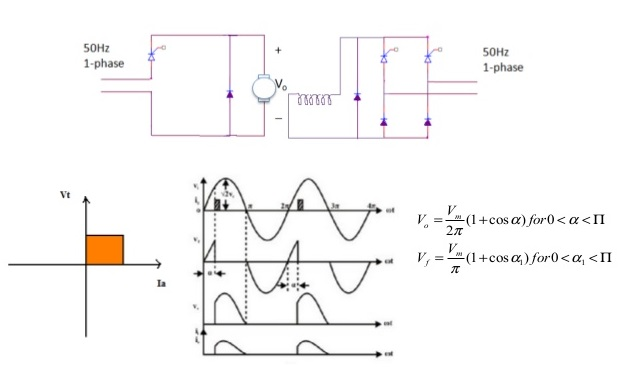## Search This Blog

### Single phase half wave converter drives

• A single phase half wave converter drive a shown in the figure. A separately excited dc motor, fed through single phase half wave converter, as shown in the figure.
• In this converters, motor field circuit is fed through a single phase semiconductor in order to reduce the ripple content in the field circuit.
• So in a single phase, half wave converter feeding a dc motor offers one quadrant drive.
• For a single phase half wave converter, average output voltage of converter the voltage V = armature terminal voltage. Vt is given by,
V0 = Vt = V/ 2∏ * ( 1+  Cos ɖ )    for 0 < ɖ <  ∏

Where Vm = maximum value of the source voltage

For single phase semi converter in the field circuit, the average output voltage is given by

Vf   =  V/ ∏ * ( 1+  Cos ɖ )  0 < ɖ1  <Single phase half wave converter drive
• A single phase half wave converter drive a shown in the figure. A separately excited dc motor, fed through single phase half wave converter, as shown in the figure.
• In this converters, motor field circuit is fed through a single phase semiconductor in order to reduce the ripple content in the field circuit.
• So in a single phase, half wave converter feeding a dc motor offers one quadrant drive.
• For a single phase half wave converter, average output voltage of converter the voltage V = armature terminal voltage. Vt is given by,
V0 = Vt = V/ 2∏ * ( 1+  Cos ɖ )    for 0 < ɖ <  ∏

Where Vm = maximum value of the source voltage

For single phase semi converter in the field circuit, the average output voltage is given by

Vf   =  V/ ∏ * ( 1+  Cos ɖ )  0 < ɖ1  <Single phase half wave converter drive